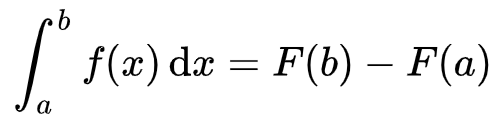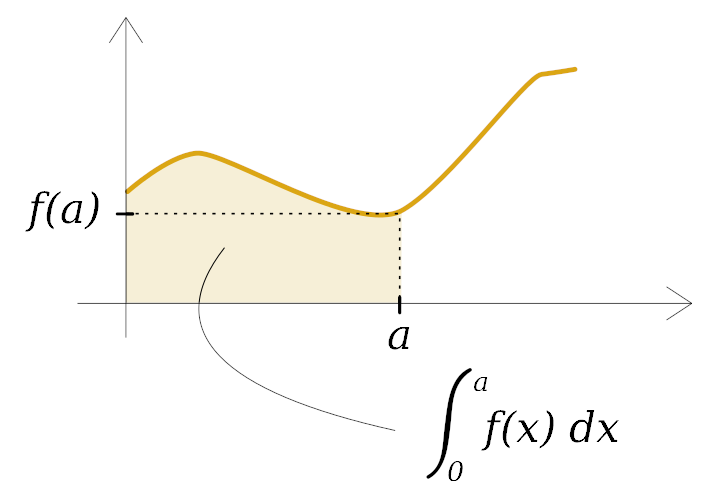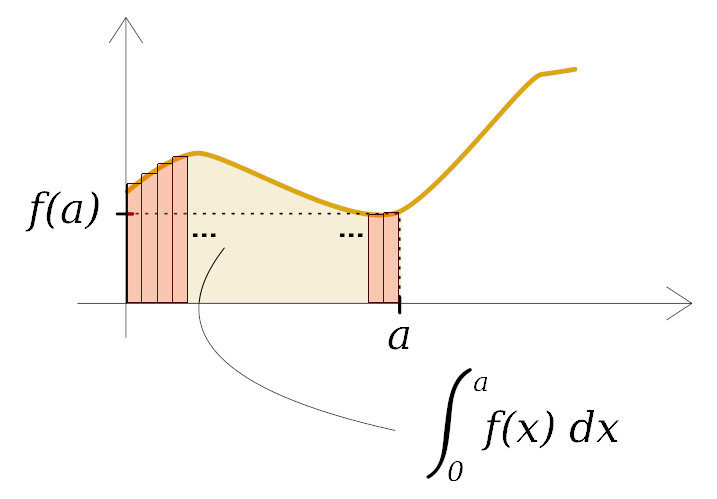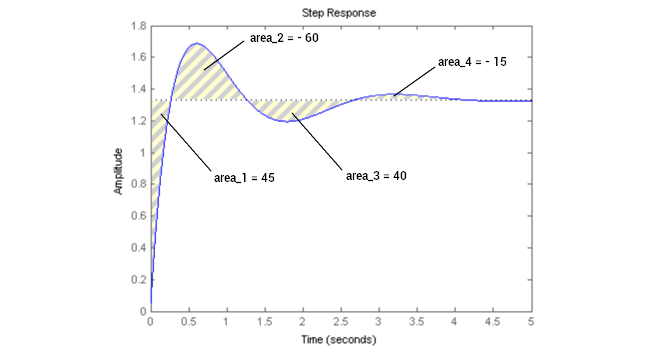# PID: The I, as in integral

We go on with the PID letters. This post deals with the I. You can visit the first posts of this series, the introduction to PID, and the proportional post.

In the previous post, I introduced to you what was a PID control and how the P — for proportional — was working in it. This post is about the I of PID.

Welcome to the I_-_post.

As you can remember from my previous posts, a PID is most of the time a coded feedback loop control working into a system that includes at least:

• a powered actuator (e.g. a DC motor),
• a sensor (e.g. a speed sensor), and,
• the associated command (most of the time an electronic board with a microprocessor).

A PID is a tool used for politely but firmly asking a machine to move or react precisely the way you want while adapting to its changing environment.

The changing environment can be, for example, a dynamic charge or something slowing the rotation.

I remind you of the must-known vocabulary you will need to go on reading:

• Setpoint: the user-defined value to be reached by the actuator (a speed, a torque, etc.).
• Process variable: the real actuator’s value, retrieved by the sensor in real-time.
• Error: the difference between the setpoint and the process variable.
• Steady-state: when the system stops oscillating and finds its full balance.

Unlike the proportional part of a PID, the integral part can’t be used alone, as it wouldn’t have any effect. Instead, the integral is either proportional (PI) or proportional and derivative (PID). The proportional part P is always used in a PID control.

Note: P, PI, PD, and PID are the four possible combinations of loop control.

Now, to the point:

## The I stands for integral because it integrates the computed error into the PID command.

What does that even mean?

In the last post, the P-post, you thought you casually took the computed error and that you multiplied it by a chosen constant, P. But what you did is that you multiplied P by the calculated last mistake. At each loop, its value changes:

• The system is running. The process variable evolves at each loop.
• However, the setpoint stays the same unless you want to change it.
• The error, which is equal to ProcessVariable - setpoint, evolves at each loop.

Then the error must not be seen as a variable anymore but as a function of time. Let’s call it ε(t).

Let’s get back to the integral thing. Integration is a mathematical operation applied to a function continuous into a given interval. Let me write the method of calculation down here, just for fun:f(x) is the function, and it’s integrated from x = a to x = b. You can express it like this: “The integral of f(x) from a to b is equal to the primitive F of f at b minus the primitive F of f at a. The primitive of a function is the inverse of the derivative, but at this point, you don’t care. We don’t want to go too deep into mathematics. Let’s say that integrating a function is like calculating the area between its representative curve and the x-axis (abscissa) and into a wanted interval where the function is defined:Thinking of area, integrating a function — although it’s not too difficult if you know how to use the method — may seem like pain: it’s like dividing the area into a bunch of really thin rectangles (the thinner, the better), calculating their area, and adding them together. It results only in an approximation because the upper side of a rectangle doesn’t quite follow a curve:There is virtually an infinite number of values stuck between the two numbers 0 and a, for example, whatever is a. So no one reasonable will accept to do the maths with almost infinite numbers of thin rectangles.
Let’s breathe a moment. There are not only maths in life. There is nature too.And today we are lucky: we are writing a PID in programming code, meaning that all the values of the error function ε(t) during a set of loops are known and can be written down in a finite number of them, as many as the times the loop iterates. That means the error function ε(t) is discretized.

No. Infinite. Number. No. Complicated. Maths.

Still, the question remains: How to integrate ε(t)?

It’s easy: You add together all the previous values of the error function. It’s like adding all the thin rectangles under the error curve.

Then you multiply the total by a constant called KI, which will allow you to control the power of an integral part. For example, if KI is very small, like KI = 0.005, the essential part will have very few effects on the command. KI, like KP, is a value you will want to set to tweak your final PID.

Here is how you want to express it: you declare a variable, let’s call it sum_e, that will iterate with each new value of the error ε(t) at each loop of the system, something like sum_e += e (e being the error at each loop), and you multiply this variable by KI in the command function. We now have this:Note: When you program a control loop feedback, at the beginning of the actual PID loop code and just after defining the error e = set_point -process_variable, the line sum_e += e is essential, so that in the following passage into the loop sum_e would evolve.

Visually, after many loops, the operations made by the integral command look like this on the system:In this example, the expression of fI(e) at t = 4.5s is fI(e) = KI.(45 -60 + 40 -15) = 10.KI

It’s almost time for you to implement an integral part of your code. But before you do that, let’s figure out the effect of an integral part on a feedback loop control.

Don't hesitate to start with Luos and discover our tutorials by following our get started step by step!

## The effects of I

With an integral part, it’s as if the command had a memory: it knows the previous errors that were made. And the bigger the sum of the previous errors (sum_e), the bigger fi(e). The bigger fi(e), the stronger the command. So if the past errors were too important before, it’s time to correct them with a bigger command.

• ε(t) being a function of time, you can see that every single value it took in the past loops affects an integral part of the PID:

The integral looks into the past of the system.

• Adding an integral command will correct the static error induced by a proportional command set alone. Indeed, as long as there is an error, i.e., ε(t) ≠ 0, even if ε(t) is small enough to have no effect on the proportional part, it will still impact the system with its residual value.

You now have two parameters to tune, KP and KI. You can program a proportional control for your system and refine it with an integral control, making it a PI.

In the next post, we will see the last letter of PID, the D.

Get Started with Luos

Tags: